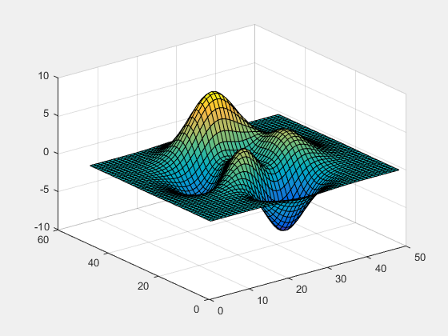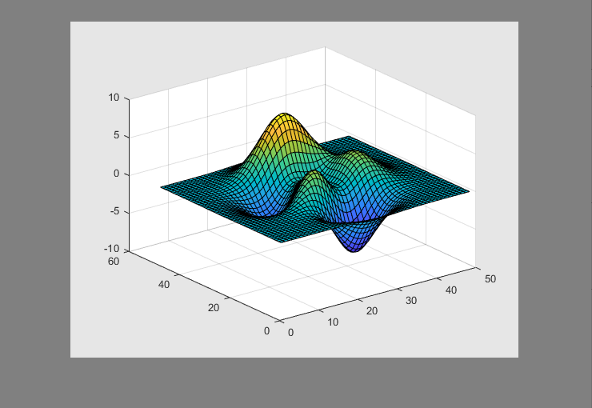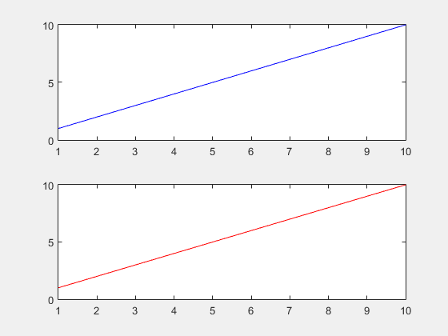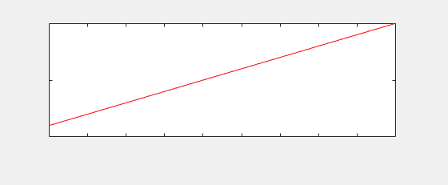getframe

说明

F = getframe 捕获显示在屏幕上的当前坐标区作为影片帧。F 是一个包含图像数据的结构体。getframe 按照屏幕上显示的大小捕获这些坐标区。它并不捕获坐标区轮廓外部的刻度标签或其他内容。

F = getframe(ax) 捕获 ax 标识的坐标区而非当前坐标区。

F = getframe(fig) 捕获由 fig 标识的图窗。如果您需要捕获图窗窗口的整个内部区域（包括坐标区标题、标签和刻度线），则指定一个图窗。捕获的影片帧不包括图窗菜单和工具栏。

F = getframe(___,rect) 捕获 rect 定义的矩形内的区域。指定 rect 作为 [left bottom width height] 形式的四元素向量。将此选项用于上一语法中的 axfig 输入参数。

示例

plot(rand(5))
F = getframe;F 是一个结构体，其中包含的 cdata 字段中包含捕获的图像数据。

figure
imshow(F.cdata)surf(peaks)
F = getframe(gcf);F 是一个结构体，其中包含的 cdata 字段中包含捕获的图像数据。

figure('Color',[0.5 0.5 0.5])
imshow(F.cdata)plot(rand(5))drawnow
ax = gca;
ax.Units = 'pixels';
pos = ax.Position
pos =

73.8000   47.2000  434.0000  342.3000

marg = 30;
rect = [-marg, -marg, pos(3)+2*marg, pos(4)+2*marg];
F = getframe(gca,rect);
ax.Units = 'normalized';

figure('Color',[0.5 0.5 0.5])
imshow(F.cdata)plot(rand(5))
xlabel('x values')
title('Plot of Random Data')drawnow
ax = gca;
ax.Units = 'pixels';
pos = ax.Position;
ti = ax.TightInset;

rect = [-ti(1), -ti(2), pos(3)+ti(1)+ti(3), pos(4)+ti(2)+ti(4)];
F = getframe(ax,rect);

figure('Color',[0.5 0.5 0.5])
imshow(F.cdata)tiledlayout(2,1)
ax1 = nexttile;
plot(1:10,'b')
ax2 = nexttile;
plot(1:10,'r')F = getframe(ax2);

figure
imshow(F.cdata)Z = peaks;
surf(Z)
axis tight manual
ax = gca;
ax.NextPlot = 'replaceChildren';

loops = 40;
F(loops) = struct('cdata',[],'colormap',[]);
for j = 1:loops
X = sin(j*pi/10)*Z;
surf(X,Z)
drawnow
F(j) = getframe(gcf);
end

fig = figure;
movie(fig,F,2)

输入参数

getframe 捕获包围坐标区轮廓的最小矩形中的内容。如果您要捕获所有刻度值和标签，则改用 fig 输入参数。

输出参数

• cdata - 存储为 uint8 值数组的图像数据。图像数据数组的大小取决于您的屏幕分辨率。

• colormap - 颜色图。在真彩色系统中，此字段为空。

• 如果您查询 getframe 捕获的区域（图窗、坐标区或由 rect 指定的区域）的大小，则以像素为单位的大小可能与 cdata 中的元素数不匹配。这种差异是因为 cdata 中的元素数取决于您的屏幕分辨率（以及操作系统设置），而 MATLAB® 中的像素数可能不对应于屏幕上的实际像素数。

• 从 R2015b 开始，如果您使用高分辨率系统，则 cdata 的大小可能大于在以前版本中或在其他系统上的大小。

局限性

• MATLAB Online™Web Apps (MATLAB Compiler) 中，getframe 不支持以下功能：

• 捕获使用 uifigure 函数创建的图窗的内容或图窗中的任何坐标区。

• 捕获使用 App 设计工具创建的 App 的内容或 App 中的任何坐标区。

详细信息

像素

• 在 Windows 系统上，一个像素是 1/96 英寸。

• Macintosh 系统上，一个像素是 1/72 英寸。

提示

• 为了在使用 getframe 值获得最快的性能，请确保图窗在屏幕上可见。如果图窗不可见，getframe 仍可捕获该图窗，但性能可能会减慢。

• 为了更好地控制图像数据的分辨率，请改用 print 函数。带有 print 的 cdata 输出参数返回图像数据。resolution 输入参数控制图像的分辨率。